# Characteristic equation with complex roots

### What is a complex root

In the last lesson we talked about real and distinct roots for those characteristic equations in which the discriminant was equal to a positive value. But remember, for second order constant coefficients linear homogeneous differential equations that get transcribed into characteristic quadratic equations there are three possible type of roots as an outcome: real distinct roots, complex roots and repeated roots.

 Discriminant Roots $B^{2}-4AC = positive$ $or$ $B^{2} > 4AC$ Real and distinct roots $B^{2}-4AC = negative$ $or$ $B^{2} < 4AC$ Complex roots $B^{2}-4AC = 0$ $or$ $B^{2} = 4AC$ Repeated roots

We will focus now on the second case: complex roots, in which we find the discriminant equal to a negative value. Remember the discriminant formula consists of the terms inside the square root in the quadratic formula, which means that if the discriminant is negative we will be using square roots of negative numbers which takes us in the realm of imaginary numbers, and so let us start by explaining what imaginary numbers are all about.

An imaginary number is any number which contains a multiplication of a real number and the imaginary unit, where this unit imaginary number is defined as the solution to the equation $x^{2}+1=0$ or simply the square root of -1 and represented as $i$. The imaginary numbers arised from the need to represent and work with the square roots of negative numbers which do not exist in real numbers, and so, since they are not "real" they got called "imaginary" (Thumbs up for those creative mathematicians and the names they come up with! *wink*).

With that in mind, now let us look into complex numbers:

A complex number is that which has at least one real and one imaginary term as shown in equation 1. Notice that in equation 1 both a and b are real numbers while $i$ represents the imaginary unit, but even though b in itself is real, it is in the imaginary part of the complex number. And so, when having a complex number such as $z = 4 + 2i$ (where a=4 and b=2), we say the real part of the complex number z is $Re(z) = a = 4$ and the imaginary part of the complex number is $Im(z) = b = 2$. These Re(z) and Im(z) form an ordered pair (Re(z),Im(z))=(a,b)=(4,2) that help us represent the complex number in a graphic plane that we call, the complex plane.

The complex plane is a graphic representation to denote complex numbers, in other words, is a geometric plane where we can graph both real and imaginary numbers. The complex plane looks very similar to the typical first quadrant of a cartesian plane but in this case the two axes are called the real and imaginary axes instead of being x and y. The horizontal axis represents the real axis and the vertical one is the imaginary axis. The complex plane is also called the z-plane since is the representation of numbers in terms of equation 1

Going back to the topic of roots, what are complex roots exactly?

Well, if we take a look at what is the discriminant for our lesson of today, it turns out that while trying to find the solution for the characteristic equation from a second order constant coefficients linear homogeneous differential equation we will end up with a negative number inside the square root in the quadratic formula, and therefore our results will be a set of two complex solutions for r. In other words, our roots will each be a complex number, and thus the name of complex roots.

These computations will be shown in detail throughout the next section in our lesson, we will first show how to solve second order differential equations that produce complex roots in a complete clear manner relating our process with the one found during the lesson about real distinct roots. Actually, we will use what we know from that past lesson to develop an efficient way to look for complex roots and find the particular solution to the differential equation faster, and so, expect a long computation throughout the very first part of next section; afterwards a list of steps to follow will be provided and we will end the lesson with some final examples which will be done using a summarized version of the process in order to maximize time when dealing with multiple problems at once.

Although most of these concepts and the process you are about to see are related to the lesson right before this one, we recommend that if it has been awhile since you last studied these topics you go back and check a few lessons before continuing to our next section. Besides the lesson on the real distinct roots, we recommend you to take a look into the lessons about the quadratic function in general form, quadratic equations, the quadratic formula, the discriminant, solving quadratic equations by completing the square, etc so you are prepared for what is coming next.

### How to solve second order differential equations

Having a second order constant coefficients linear homogeneous differential equation of the form: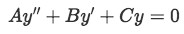Equation 2: General form of a second order constant coefficients linear homogeneous differential equation

We learned on our lesson about solving differential equations with real distinct roots that we can solve such equations in a straightforward and efficient manner by transcribing them as algebraic expressions which lead our path to a solution using simple algebra. Such algebraic expressions emulating the form of the second order constant coefficients linear homogeneous differential equation are what we call a characteristic equation, which has a general form of:

How to find complex roots:

For the case of complex roots we have already mentioned that a complex number comes about when you have a number composed of a real part and an imaginary part. Such imaginary terms usually result from the computation of square roots of negative numbers and so, that is exactly how we could end up with a differential equation which contains a complex root.

In simple terms, when solving for the characteristic equation on these kinds of problems we will end up using the quadratic formula while finding the root, this equation contains a square root term which will consist of a square root operation of a negative number and thus resulting in a set of complex numbers as the results for r.

The operation described above can be noted in the next formulas, where you can observe that the particular case for complex roots arise from us plugging the constant coefficients values into the quadratic formula in order to obtain the set of roots for r and finding out that the square root in the equation contains a negative number result. This negative number comes from the fact that in such cases the coefficient B squared is smaller in quantity than the multiplication of A and C by 4 as shown in table 1, and it goes as follows:

And so we observe that whenever we find a case in which we find the condition $B^{2} < 4AC$ it means our problem is solving a differential equation with complex roots. Our resultant r will be a complex number with two components: the real term denoted in the equations above as "Re(r)" (meaning "real of r") and named $\lambda$; and the imaginary term denoted as "Im(r)" (meaning "imaginary of r") which is named $\mu$.

Our set of roots in this case will look like:

Having learned all this about solving differential equations with complex roots is time for us to come up with a formula to facilitate the process of finding a general solution to these type of equations. And so, for the next paragraphs we will be simply working around with known equations in order to come up with this final general solution formula that will help us out in solving these problems.

We start by using the formula for the general solution of a differential equation with real distinct roots: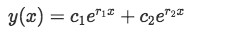Equation 6: General solution equation for a second order differential equation with real distinct roots

Notice that we have a set of two roots that we can plug in the places for $r_{1}$ and $r^{2}$ and so we can simply use equation 6, plug in our r's from equation 5 and just follow through simplifying that equation until we come up with a straightforward form of a general solution formula for our problems with complex roots: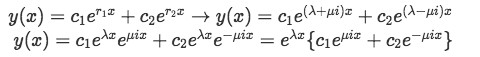Equation 7: Solving for the general solution of differential equations with complex roots (part 1)

In order to continue the simplification of the general solution formula we are looking for, we make use of a fundamental equation from complex mathematical analysis called the Euler's formula. This formula describes the relation of a complex exponential function and the basic trigonometric functions of sine and cosine as follows:

We plug this into our computations from equation 7 and continue the simplification process: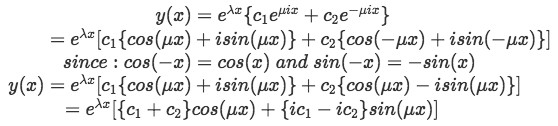Equation 9: Solving for the general solution of differential equations with complex roots (part 2)

Now we just simplify the equation by merging together the unknown constants and renaming them: $K_{1} = (c_{1} + c_{2})$ and $K_{2} = (ic_{1} - ic_{2})$ so we obtain the general solution expression for differential equations with complex roots: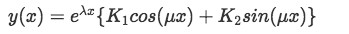Equation 10: Final general solution expression for differential equations with complex roots

Equation 10 is your go-to equation when solving these kind of problems, although the computations shown above seem extensive and a bit complicated, the method of solving a differential equation with complex roots has been simplified for you now that you have the general solution form equation. Still we will work through our first example following the complete methodology so you can see how we went from the general solution form for equations with real distinct roots, to transform it to solve problems with complex roots.

Example 1

Find the particular solution to the following differential equation with initial conditions y(0)=0 and y'(0)=2.

• We first obtain the characteristic equation:
• Where the constant coefficients are A=1, B=-2 and C=2. Then finding complex roots using the quadratic formula:
• Now we use equation 6 in order to find the general solution of the differential equation. This time we will be using this equation to demonstrate how the process starts the same as for those problems with real distinct roots but then it simplifies into other general solution form to comply with the usage of complex numbers: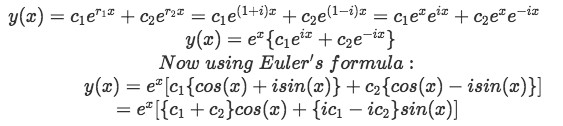Equation for example 1(c): Solving for the general solution to the differential equation
• Where we merge and rename the constants: $\{c_{1}+c_{2}\} = K_{1}$ and $\{ic_{1}-ic_{2}\} = K_{2}$ in order to obtain the final expression for the general solution to the differential equation:
• Now is just a matter of us computing the first derivative y' of the general solution y, and apply the initial conditions of y(0)=0 and y'(0)=2 to both, y and y', in order for us to find the values of the unknown constants $K_{1}$ and $K_{2}$.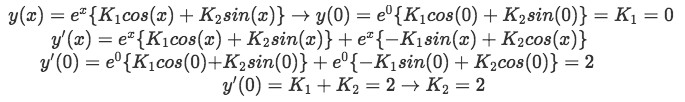Equation for example 1(e): Finding the values for the unknown constants
• Now we plug the values to our general solution found in equation for example 1(d) and this gives us the particular solution the the differential equation in our example 1:

After solving this past problem, we remind you this method can be summarized and solved in a faster way by skipping a few of the computations above, therefore, we have listed the steps for the whole simplified process to follow in the next paragraphs and will be solving the next examples using this summarized method.

Steps to find the particular solution to a second order constant coefficients linear homogeneous differential equation with complex roots:

1. Given a differential equation in the form of equation 2, the first step is to find its characteristic equation in the form of equation 3.
1. Identify the constant coefficients A, B and C.
2. Solve the quadratic equation and find the set of complex roots using the quadratic formula (as shown in equation 4).
1. Identify $\lambda$ and $\mu$. Notice that $\mu$ does not have a sign associated with it because it has already been taken care of with the signs worked up through the trigonometric identities in equation 9, therefore, $\mu$ should be just a number while $\lambda$ may or may not have a negative sign.
3. Plug the values of $\lambda$ and $\mu$ into equation 10 to obtain the general solution to the differential equation.
4. Obtain the first derivative of y and apply the initial conditions to both y and y' in order to find the values for the two unknown constants in the general solution.
5. Plug the found values of the unknown constants into the general solution and obtain the particular solution to the differential equation.

This process should take much less time that what we have done so far, so let us put it into practice by solving the next two examples of solving second order differential equations which contain imaginary roots:

Example 2

Obtain the particular solution to the given second order differential equation using the initial conditions: $y(\frac{\pi}{2})$ = -1 and $y'(\frac{\pi}{2})$ = 1.

• Step 1:
Find the characteristic equation:

Where A=1, B=4 and C=8.

• Step 2:
Find the set of complex roots using the quadratic formula and identify $\lambda$ and $\mu$.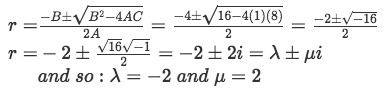Equation for example 2(b): Solving for the complex roots and identifying lamda and mu.
• Step 3:
Plug the values of $\lambda$ and $\mu$ into equation 10 to obtain the general solution to the differential equation:
• Step 4:
Obtain the values of the unknown constants by computing the first derivative of y and applying the initial conditions of $y(\frac{\pi}{2})$=-1 and $y'(\frac{\pi}{2})$=1 to both y and y':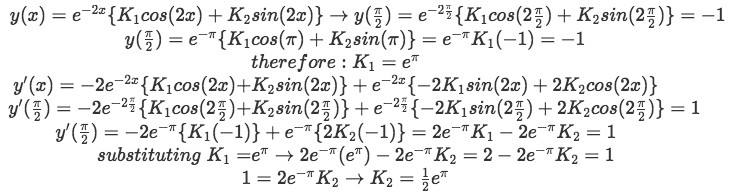Equation for example 2(d): Finding the values of the two unknown constants
• Step 5:
We plug the found values of the unknown constants into the general solution equation and obtain the particular solution to the differential equation:

Example 3

Using the initial conditions of y(0)=4 and y'(0)=5, compute the particular solution to the second order constant coefficients linear homogeneous differential equation below:

• Step 1:
Find the characteristic equation:
Where A=1, B=-2 and C=4.
• Step 2:
Find the set of complex roots of the characteristic equation using the quadratic formula and identify $\lambda$ and $\mu$.
• Step 3:
Plugging the values of $\lambda$ and $\mu$ into equation 10 we find the general solution to the differential equation:
• Step 4:
Now we calculate the values of the unknown constants by obtaining the first derivative of y and applying the initial conditions of y(0)=4 and y'(0)=5 to both y and y':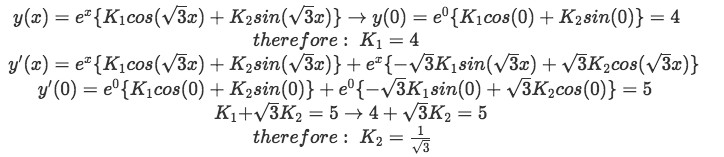Equation for example 3(d): Finding the values of the two unknown constants
• Step 5:
And so, the particular solution is:

If you are interested in continuing your studies and practice on problems solving differential equations with complex roots, we suggest you to visit these notes on differential equations where you will find a few more problems and somewhat different methodology that will help you out by give you a glimpse of different perspectives while solving these type of problems.

### Characteristic equation with complex roots

#### Lessons

Complex Numbers and Euler's Formula:

A complex number is a number of the form:

$z=a+bi$

We can plot these numbers on the complex plane: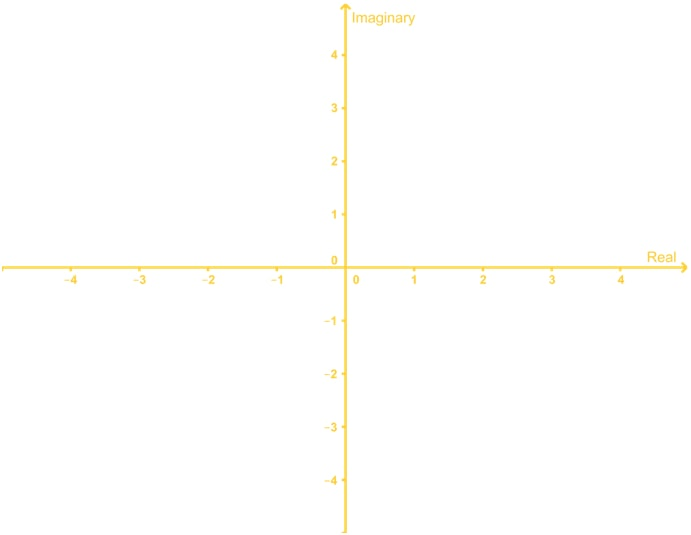Euler's Formula:
$e^{i \theta}=\cos (\theta) + i\sin(\theta)$

Characteristic Equation with Complex Roots:

In the previous section we came up with a method to solve linear homogeneous constant coefficient second order differential equations:

$Ay''+By'+Cy=0$

By using the characteristic equation:

$Ar^2+Br+C=0$

$r=\frac{-B\pm\sqrt{B^2-4AC}}{2A}$

Let's suppose that $B^2$ < $4AC$. Hence we're in the realm of complex roots.

$r=\frac{-B\pm\sqrt{B^2-4AC}}{2A}$ $=\frac{-B}{2A} \pm \frac{\sqrt{B^2-4AC}}{2A}$

Or alternatively:
$r=\lambda \pm \mu i$
$r_1=\lambda + \mu i$
$r_2=\lambda - \mu i$

And the general solution will be:

$y(x)=e^{\lambda x} ((c_1) \cos ( \mu x)+(c_2)( \sin (\mu x))$
• Introduction
a)
A very brief run-down on Complex Numbers and Euler's Formula

b)
Using the Characteristic Equation with Complex Roots

• 1.
Determining Complex Solutions to the Characteristic Equations
Find the particular solution to the following differential equation:

$y''-2y'+2y=0$

With initial values $y(0)=0, y' (0)=2$

• 2.
Find the particular solution to the following differential equation:

$y''+4y'+8y=0$

With initial values $y( \frac{\pi}{2} )=-1$, $y' (\frac{\pi}{2})=1$

• 3.
Find the particular solution to the following differential equation:

$y''-2y'+4y=0$

With initial values $y( 0)=4$, $y' (0)=5$